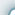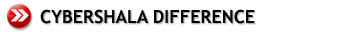AdvantageWith us, you don't learn just one process, as you do in more traditional establishments. You learn many different ways to approach a problem. Here's an analogy: think of all the times you're stuck in a traffic jam due to an accident on a large highway. If you're a local, you can probably get out of the jam at the next exit and to your destination without much hassle because you know several ways to get anywhere. If you're from out of town and without a map, you're stuck, because you only know that one route. With cybeRShala, you become a local in the land of math; with traditional programs, you're a tourist. Here's how we do it. Our Learning Facilitators, combined with the wisdom of the Singapore Math Curriculum ensure: Visual representation of abstract concepts: in early grades – e.g. 21-9 (we visually decompose one 10 into ten 1s and show the meaning of this abstract calculation). Mental calculation techniques: are stressed and imparted from early grades e.g. 81-25 is learned to be solved like81 = (31 + 50) – 25 = (50-25) + 31 = 25 +31 = 56 Identify relationship between topics: e.g. how ratio, percent, fractions and decimals are related1:2 = 50% = ½ = 0.5 Reasoning and rational behind formulas: e.g. why is the circumference of a circle = pi times diameter, why is the area of triangle = 1/2 * b * h Meaning of math concepts: e.g. dividing a whole number by a fraction say 3 divided by ½. If you had 3 oranges and divide each in half how many pieces would you have6. Teach Math comprehension: After a skill is taught, children work on challenging problems designed to do a few things: They look at the new concept from different angles. They're complicated, requiring several operations to solve them. They require skills the students learned in previous lessons, thus reviewing old work while practicing new work. Challenging story problems: Require multiple steps, and the steps often review something you studied in a prior lesson. A conventional American math program might ask, "What is the perimeter of a rectangle with a height of 12 inches and a width of 8 inches?" Compare that to a Singapore Math problem, which might ask, "A rectangle has a height of 12 cm and a width of 8 cm. A square has the same perimeter as the rectangle. What is the height of the square?" Can’t get away with memorizing algorithms: You can solve a few problems by following the pattern given in the text, but pretty soon the recipe-follower is in over his head. You HAVE to think the problems through, think through what is really going on to solve most of the problems. They are like puzzles and require mathematical thinking. Model drawing: without a picture-drawing approach to solving these challenging problems, one has to either use algebra or give up completely. It's a great feeling to solve these problems (without algebra), and it gives students a real sense of accomplishment. Our Goal: To gradually and systematically help children view their skills as tools which can be used to solve a wide variety of problems. Eventually, we want them to look at unfamiliar problems and try to figure out which of their tools they can adapt to a situation.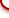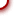cybeRShala Difference Lesson Illustration How It Works Compare Us Request Info Placement Test Free Trial Tell a friend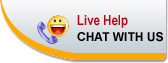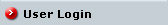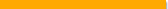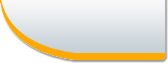Home | FAQ | Tell a Friend | Contact Us | Compare Us| Homeschool Resources | Link To Us | Sitemap cybershala.com Inc. All Rights reserved | Terms of service | Privacy policy